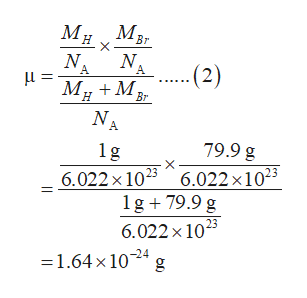# Using the rigid rotor model, calculate the energies in Joules of the first three rotational levels of HBr, using for its moment of inertia I = μR2, with μ = mHmX/(mH + mX) and equilibrium internuclear distance = 1.63 Å. To put these energies into units that make sense to us, convert energy to kJ/mol. (Simply estimate atomic masses from the average atomic weights of the elements given in the periodic table).

Question
1 views

Using the rigid rotor model, calculate the energies in Joules of the first three rotational levels of HBr, using for its moment of inertia I = μR2, with μ = mHmX/(mH + mX) and equilibrium internuclear distance = 1.63 Å. To put these energies into units that make sense to us, convert energy to kJ/mol. (Simply estimate atomic masses from the average atomic weights of the elements given in the periodic table).

check_circle

Step 1

Rotational energy (E) for one mole of HBr is calculated by equation (1) in which ‘h’ is the plank constant, I is the inertia, NA is the Avogadro number and J is the rotational quantum number.

Step 2

The reduced mass (μ) for the molecule is calculated by equation (2) in which MH is the mass of hydrogen in gram, MBr is the mass of bromine in gram and NA is the Avogadro number. The reduce mass for the HBr molecule is 1.64 ×10-24 g.help_outlineImage TranscriptioncloseMBr Мн A - (2) A M M NA Br 79.9 g 1g 6.022 x 1023 6.022 x 1023 1g 79.9 g 6.022 x 1025 1.64x1024 fullscreen
Step 3

The reduced mass 1.64 ×10-24 g of HBr in kilogram is e...

### Want to see the full answer?

See Solution

#### Want to see this answer and more?

Solutions are written by subject experts who are available 24/7. Questions are typically answered within 1 hour.*

See Solution
*Response times may vary by subject and question.
Tagged in

### Quantum mechanics